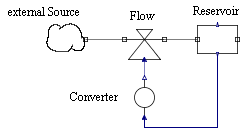# Introduction to System Dynamics

• Simulations in biology, ecology or economy:
• highly complex systems
• causal relationships often unclear
• simulation as testbed to check new ideas
• mathematical formulation
• usually as differential equation
• often too abstract for users
• functional relationships often based on empiric data (tables, plots)
• graphical modeling with system dynamics diagramms
• emphasis on causal relationships
• only very few general blocks
• mathematical relations are hidden, equations are defined as parameters
• several commercial modeling and simulation environments, e. g. Stella, Vensim, Simile
• invented by Jay Forrester around 1955
• Basic building blocks of system dynamics diagrams:
• Reservoir (or stock)
• corresponds to a state variable
• needs initial value
• Flow
• defines rate of change (positive/negative) of a reservoir
• connects reservoir with another reservoir or external sources/sinks (cloud)
• is symbolized as a valve
• Converter
• external parameter or auxiliary variable
• computed using other values
• concrete computation is hidden as parameter
• Connector
• specifies, which variables affect others
• graphical representation
•• Physical modeling:
• models built from "physical" components (masses, resistors, valves) instead of integrators or function blocks
• connecting lines represent "physical" connections (flanges, wires, pipes) instead of signals
• internal representation uses Modelica language
• object-oriented, equation-based modeling language
• provides means for graphical representation of components and models
• huge free library of components (MSL = Modelica standard library)
• simulation
• equations come from components and connections
• automatically combined, simplified (highly non-trivial!) and numerically solved
• modeling and simulation environment
• Modelica library SystemDynamics.mo:
• based on SystemDynamics 2.0 by Cellier
• design changed to cope with blocks like Oven
• using library in OpenModelica
• start OMEdit
• load base library SystemDynamics.mo und examples library SystemDynamicsExamples.mo
• both are displayed in Libraries pane
• design of base library
• packages Reservoirs, Flows and Converters
• predefined components for common equations
• user-defined components necessary for special mathematical relations
• definition of such components easy
• packages Interfaces contains supporting auxiliary components
• design of example library
• Examples contains executable models
• packages Examples for executable models,  AuxComponents for auxiliary components
• required data sets in package Resources
• Simple growth model Inflow:
• only one state variable
• growth (inflow)
• defined by flow (valve symbol)
• rate variable (i. e. amount/time)
• defined by ConstantConverter
• diagram
•• Building the model:
• pick up components from library pane and drag them into model pane
• form Reservoirs: Stock, CloudSource
• from Flows: Flow
• from Converters: ConstantConverter
• connect components
• set parameter values (after double click on a component)
• initial value of reservoir (Stock): m0 = 2
• inflow rate (ConstantConverter): constValue = 0.5
• Running simulation:
• check model
• setup and run simulation
• Stop Time = 10
• automatically runs simulation
• → one output window is shown
• change window size (plot window icons "hidden" top-right)
• choose variable: Inflow.stock1.out1
•• use switch at bottom-right to retrun to model pane
• Model Outflow:
• mirror image of Inflow
•• initial value m0 = 4
• simulation
• stock value becomes negative
• is a negative level meaningful?
• alternative: use SaturatedStock with  minLevel = 0
• result
•